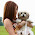## Monday, March 24, 2014

### The Quadratic Formula

A couple of weeks ago, one of my students casually mentioned that they'd learned the quadratic formula last year when I was using the ± sign.  "Great," I thought, "there goes the point of teaching the other methods of solving quadratics if the last teacher just went straight to the formula."  Given that quadratics are in no way part of the curriculum for their last class, I was perplexed.  I finally got a little more background today.  Apparently they did it "one day" and were told they "didn't have to memorize the formula," though one of my girls did have it memorized!

All that is to say that last week we studied solving quadratic equations by factoring and by square roots.  Those methods went pretty well.  Then we did completing the square.  If you haven't ever introduced it as a puzzle using Algebra tiles, you are missing the hook you need.  The intro to completing the square goes something like this:

Alright everyone, we're going to play a little game.  I need you to please take out an x squared tile and 2 x tiles.  You may add ones tiles if you want.  Please make a square.

The kids make a square by adding one.

Great.  Let's notice the area of the square and the side lengths and note that on our recording sheet. (Use graph paper here to make the diagrams better).

Next, try the same kind of puzzle with one x squared tile and 4 x tiles.  Kids will add on 4 ones.

Repeat as needed with other even numbers of x until you have success (or you run out of tiles!)

Would you build a square from one x squared tile, 5 x tiles, and ones tiles?   Disaster will strike.  Kids will build you rectangles, convinced they are squares until you suggest they measure.  Other kids will claim it's not possible.  Still others will add negative x tiles in an attempt to make it work.  Whining will most likely ensue.

So, why isn't this one working?  Let's see what you have tried.  Build some unsuccessful models, talk about why they fail.  Did you start with this model?  Build rectangle (x+2)(x+3).  Most kids will say "yes."  Why didn't it work?  They'll answer that the sides were different lengths.  So if this side is longer, could I move a tile to the opposite side?  No?  That makes the same thing?  Hmm...if we start building this with the idea of putting equal x tiles on each of two sides of the x squared tile, what will happen?  We'll have one left over.  How could we share it equally?  There might be trouble here if your tiles are plastic like mine.  They were stuck on the idea that they couldn't physically destroy my tiles.  One of the kids saw the idea of dividing that tile.  We drew the (messy) model of (x+2.5)^2.  We made the connection (easily, even) that the b value divided by two and squared provided the c value.

Once we got that far, kids understood the "what should we add to both sides to make a perfect square" aspect of completing the square, which is the only part that is really different from what they already know.  My kids have turned out to be pretty confident at completing the square.  Today, some of them got to the end of the kite relay.  The last problem was completing the square of the standard form of a quadratic equation.  I actually had three or four groups get it.  The hardest part for almost every group: adding fractions and remembering to get a common denominator.  Oh, how we forget the foundational things!

We wrote it up formally in our notes after lots of messy whiteboarding.  The kids' first reaction: "You make it look so easy."  Sorry, friends!  Their second reaction: sheer joy when they realized we'd derived the quadratic equation by completing the square.  It was cute.

What's your best tip for teaching quadratics?

Mathematically yours,
Miss B

1.I haven't taught quadratics, but I remember learning it. That was way back Grade 11.

We only got seriously into algebra in Grade 9.
Then, in Grade 11, we worked through the derivation of the Quadratic Formula, its uses, applications, etc. and several problems. The textbook we used at the time was pretty good, but I don't remember its title or authors. Nowadays, there are many websites with a lot of information, including dynamic apps, videos, and much more. I am sure they could all be worked into an explanation of quadratics that makes them easy to understand.

(But we didn't touch imaginary numbers at the time. That came later.)

2.You have shared helpful article regarding Quadratic Formula, I think this formula use in different classes with different types of questions. Your blog contain so many other useful article about the Math which are helpful for the teacher or students of math. I have found a website last week which about the Math subject. I would like to share this website here.
6th Grade Math Practice